### 14.4.1.1 A double-integrator lattice

First consider the double integrator from Example 13.3. Let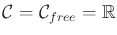and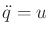. This models the motion of a free-floating particle in, as described in Section 13.3.2. The phase space is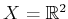, and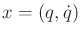. Let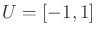. The coming ideas can be easily generalized to allow any acceleration bound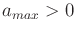by letting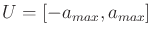; however,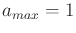will be chosen to simplify the presentation.

The differential equationcan be integrated once to yield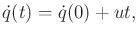(14.21)

in which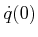is an initial speed. Upon integration of (14.21), the position is obtained as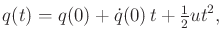(14.22)

which uses two initial conditions,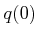and.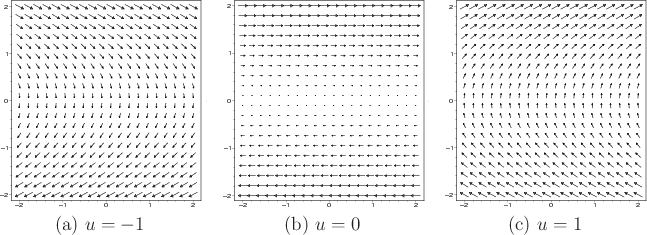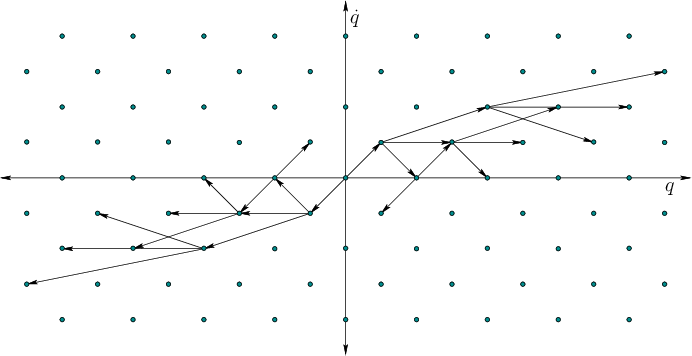A discrete-time model exists for which the reachability graph is trapped on a lattice. This is obtained by letting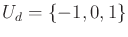and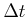be any positive real number. The vector fields overthat correspond to the cases of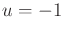,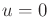, and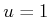are shown in Figure 14.12. Switching between these fields at everyand integrating yields the reachability graph shown in Figure 14.13.

This leads to a discrete-time transition equation of the form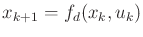, in which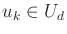, andrepresents time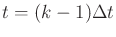. Any action trajectory can be specified as an action sequence; for example a six-stage action sequence may be given by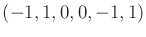. Start from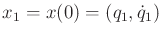. At any stageand for any action sequence, the resulting state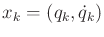can be expressed as(14.23)

in which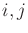are integers that can be computed from the action sequence. Thus, any action sequence leads to a state that can be expressed using integer coordinates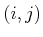in the plane. Starting at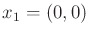, this forms the lattice of points shown in Figure 14.13. The lattice is slanted (with slope) because changing speed requires some motion. If infinite acceleration were allowed, then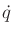could be changed instantaneously, which corresponds to moving vertically in. As seen in (14.21),changes linearly over time. If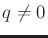, then the configuration changes quadratically. If, then it changes linearly, except when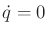; in this case, no motion occurs.

The neighborhood structure is not the same as those in Section 5.4.2 because of drift. For, imagine having a stack of horizontal conveyor belts that carry points to the right if they are above the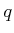-axis, and to the left if they are below it (see Figure 14.12b). The speed of the conveyor belt is given by. If, the distance traveled alongis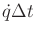. This causes horizontal motion to the right in the phase plane if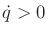and horizontal motion to the left if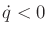. Observe in Figure 14.13 that larger motions result as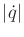increases. If, then no horizontal motion can occur. If, then thecoordinate changes by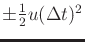. This slowing down or speeding up also affects the position along.

For most realistic problems, there is an upper bound on speed. Let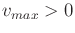be a positive constant and assume that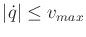. Furthermore, assume thatis bounded (all values of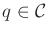are contained in an interval of). Since the reachability graph is a lattice and the states are now confined to a bounded subset of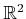, the number of vertices in the reachability graph is finite. For any fixed, the lattice can be searched using any of the algorithms of Section 2.2. The search starts on a reachability graph for which the initial vertex is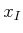. Trajectories that are approximately time-optimal can be obtained by using breadth-first search (Dijkstra's algorithm could alternatively be used, but it is more expensive). Resolution completeness can be obtained by reducingby a constant factor each time the search fails to find a solution. As mentioned in Section 5.4.2, it is not required to construct an entire grid resolution at once. Samples can be gradually added, and the connectivity can be updated efficiently using the union-find algorithm [243,823]. A rigorous approximation algorithm framework will be presented shortly, which indicates how close the solution is to optimal, expressed in terms of input parameters to the algorithm.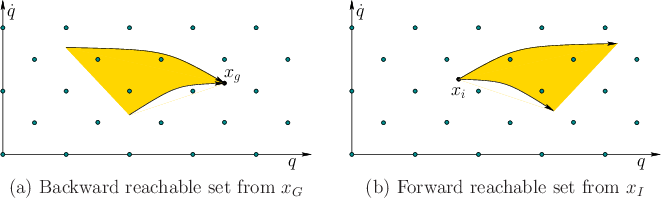Recall the problem of connecting to grid points, which was illustrated in Figure 5.14b. If the goal region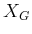contains lattice points, then exact arrival at the goal occurs. If it does not contain lattice points, as in the common case ofbeing a single point, then some additional work is needed to connect a goal state to a nearby lattice point. This actually corresponds to a BVP, but it is easy to solve for the double integrator. The set of states that can be reached from some state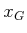within timelie within a cone, as shown in Figure 14.14a. Lattice points that fall into the cone can be easily connected toby applying a constant action in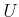. Likewise,does not even have to coincide with a lattice point. Thus, it is straightforward to connectto a lattice point, obtain a trajectory that arrives at a lattice point near, and then connect it exactly to.

Steven M LaValle 2020-08-14